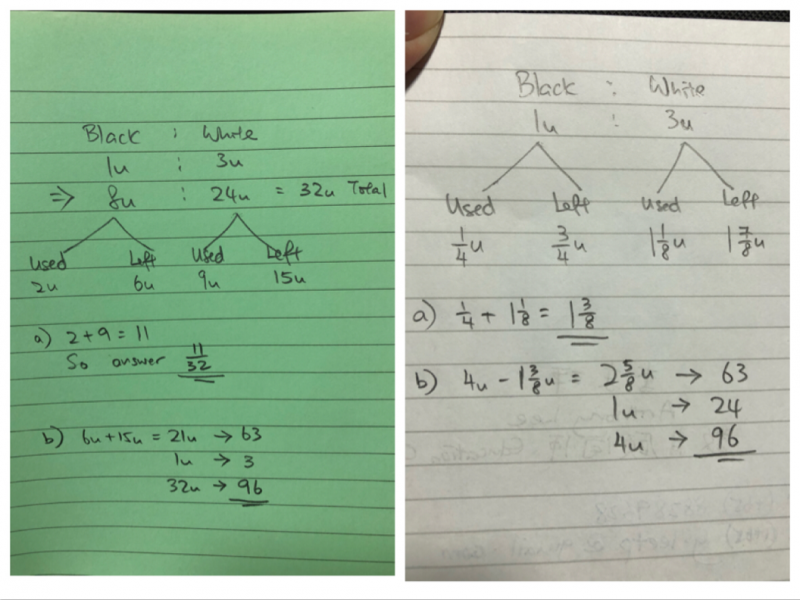# QuestionThe following is one of the PSLE Math question this year 2019. I have 2 different answers for question (a). I was told the answer for fractions cannot be more than 1 whole. Could anyone advise if both answers are acceptable or only the fraction 11/32 is accepted? Thank you very much in advance.

The number of black beads was 1/3 of the white beads at first. After Amy used 1/4 of the black beads and 3/8 of the white beads,there were 63 beads left.

a)What is the fraction of the number of beads used?
b)How many beads were there at first?

3 Answers

# Answer

“The following is one of the PSLE Math question this year 2019. I have 2 different answers for question (a). I was told the answer for fractions cannot be more than 1 whole. Could anyone advise if both answers are acceptable or only the fraction 11/32 is accepted? Thank you very much in advance.”

Whether or not the answer for a question on fractions could be more than a whole or not would depend on the actual question asked. If the answer is a fraction of a whole, then the answer obviously could not be more than a whole. For this question, only the fraction 11/32 is thus acceptable.

(a)
black beads : white beads
1 : 3
8 units : 24 units

(1/4) x 8 = 2 (black used)
8 – 2 = 6 (black left)
(3/8) x 24 = 9 (white used)
24 – 9 = 15 (white left)
2 + 9 = 11 (total used)
8 + 24 = 32 (total at first)

11/32 is the fraction of the number of beads used.

(b)
6 + 15 = 21
21 units = 63
1 unit = 3
32 units = 96

Ans : (a) 11/32 and (b) 96 beads.

1 Reply 1 Like

Thank you Mr alfretztay

1 Reply 0 Likes

You are welcome, Ms altp10.

0 Replies 0 Likes

Thank you Mr Khong Pek Mao

0 Replies 0 Likes

Quite obvious the 2nd answer for part A , i.e. 1 3/8 is wrong . Just logically speaking we can’t used a fraction of more than 1 whole. He/she needs to carry on to divide his answer with 4

1 3/8 => 11/8

11/8 divide by 4 = 11/32#

Did a short calculation, the first answer (Greenish tone) is  correct for both A and B.

1 Reply 1 Like

Thank you Mr Ender

0 Replies 0 Likes